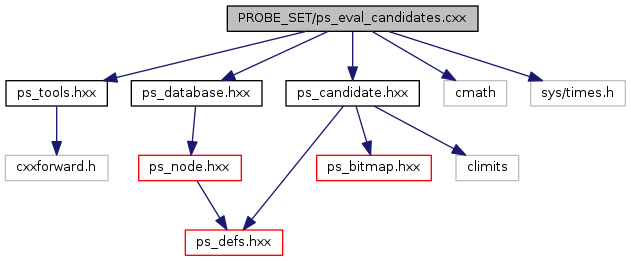ARB
ps_eval_candidates.cxx File Reference
`#include "ps_tools.hxx"`
`#include "ps_database.hxx"`
`#include "ps_candidate.hxx"`
`#include <cmath>`
`#include <sys/times.h>`
Include dependency graph for ps_eval_candidates.cxx:Go to the source code of this file.

## Typedefs

typedef set< PS_Node * > PS_NodeSet

typedef pair< PS_CandidatePtr,
PS_NodeSet
PS_Candidate2NodeSetPair

typedef multimap< unsigned
long, PS_Candidate2NodeSetPair
PS_Candidate2NodeSetPairByLengthMap

typedef set< unsigned longULSet

typedef set< unsigned shortUSSet

typedef map< float, float > FFMap

typedef set< float > FSet

## Functions

void PS_get_leaf_candidates (PS_CandidatePtr _candidate_parent, PS_CandidateSet &_leaf_candidates)

void PS_get_node_paths (PS_CandidateSet &_leaf_candidates, PS_Candidate2NodeSetPairByLengthMap &_paths)

unsigned long PS_calc_temp (const PS_ProbePtr &_probe)

void PS_calc_sums_for_nodepath (PS_NodeSet &_nodepath, ULSet &_sums)

bool PS_calc_min_sum_of_square_distances_to_average (PS_NodeSet &_nodepath, FFMap &_averages, float &_best_average, float &_min_sum)

void PS_eval_node_paths (PS_Candidate2NodeSetPairByLengthMap &_paths, float &_min_sum_of_square_distances_to_average, float &_best_average)

void PS_remove_bad_probes (PS_NodeSet &_nodes, float _average, set< unsigned int > &_probe_lengths)

int main (int argc, char *argv[])

## Typedef Documentation

 typedef set PS_NodeSet

Definition at line 34 of file ps_eval_candidates.cxx.

 typedef pair PS_Candidate2NodeSetPair

Definition at line 35 of file ps_eval_candidates.cxx.

 typedef multimap PS_Candidate2NodeSetPairByLengthMap

Definition at line 36 of file ps_eval_candidates.cxx.

 typedef set ULSet

Definition at line 72 of file ps_eval_candidates.cxx.

 typedef set USSet

Definition at line 73 of file ps_eval_candidates.cxx.

 typedef map FFMap

Definition at line 74 of file ps_eval_candidates.cxx.

 typedef set FSet

Definition at line 75 of file ps_eval_candidates.cxx.

## Function Documentation

 void PS_get_leaf_candidates ( PS_CandidatePtr _candidate_parent, PS_CandidateSet & _leaf_candidates )

Definition at line 20 of file ps_eval_candidates.cxx.

References PS_Candidate::children.

Referenced by main().

 void PS_get_node_paths ( PS_CandidateSet & _leaf_candidates, PS_Candidate2NodeSetPairByLengthMap & _paths )

Definition at line 38 of file ps_eval_candidates.cxx.

Referenced by main().

 unsigned long PS_calc_temp ( const PS_ProbePtr & _probe )
inline

Definition at line 77 of file ps_eval_candidates.cxx.

 void PS_calc_sums_for_nodepath ( PS_NodeSet & _nodepath, ULSet & _sums )

Definition at line 81 of file ps_eval_candidates.cxx.

References PS_calc_temp().

Referenced by PS_eval_node_paths().

 bool PS_calc_min_sum_of_square_distances_to_average ( PS_NodeSet & _nodepath, FFMap & _averages, float & _best_average, float & _min_sum )

Definition at line 118 of file ps_eval_candidates.cxx.

References PS_calc_temp().

Referenced by PS_eval_node_paths().

 void PS_eval_node_paths ( PS_Candidate2NodeSetPairByLengthMap & _paths, float & _min_sum_of_square_distances_to_average, float & _best_average )

Definition at line 189 of file ps_eval_candidates.cxx.

Referenced by main().

 void PS_remove_bad_probes ( PS_NodeSet & _nodes, float _average, set< unsigned int > & _probe_lengths )

Definition at line 263 of file ps_eval_candidates.cxx.

Referenced by main().

 int main ( int argc, char * argv[] )